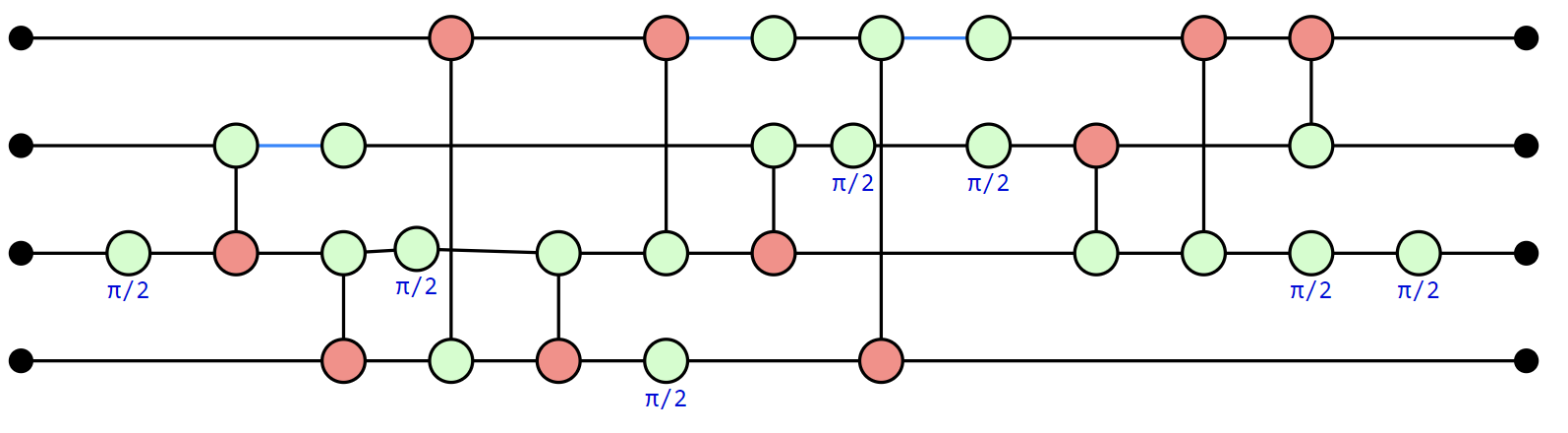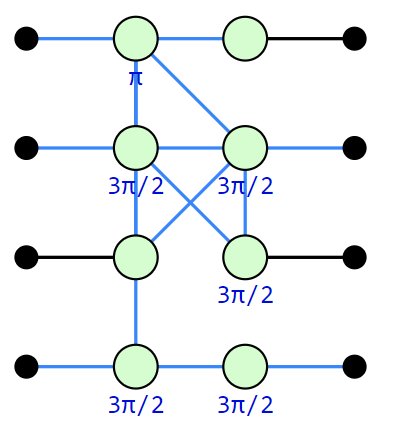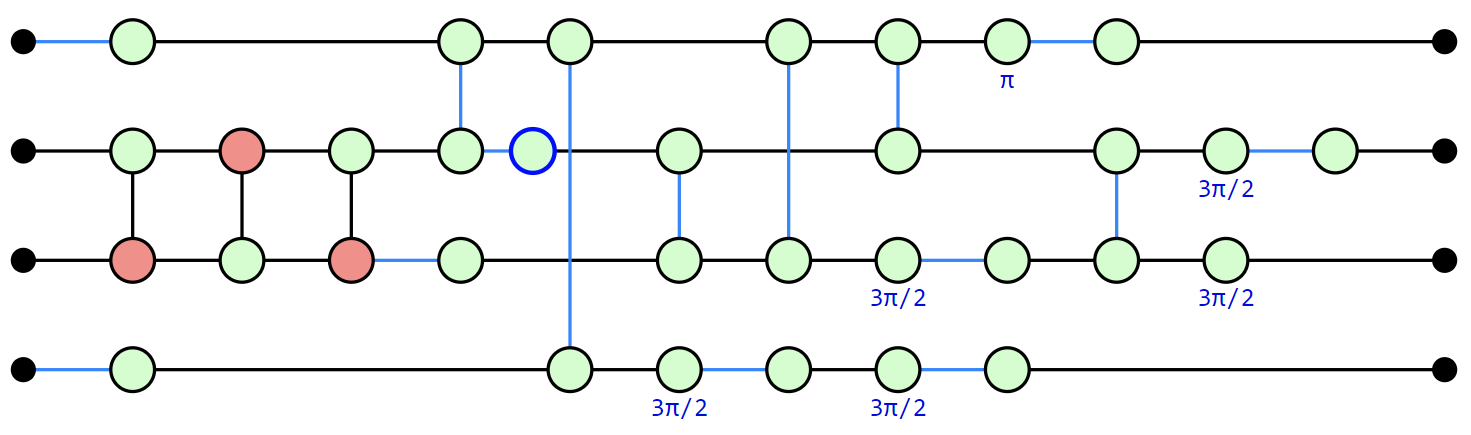# Getting Started¶

PyZX can be installed as a package using pip:

```pip install pyzx
```

If you wish to use the demo notebooks or benchmark circuits, then the repository can be cloned from Github.

The best way to get started if you have cloned the repository is to run the Getting Started notebook in Jupyter. This page contains the same general information as that notebook.

Warning

If you are using the pip installed version, please make sure it is version 0.6.0, and not 0.5.x as the api has changed considerably in between.

Warning

The newer JupyterLab as opposed to the older Jupyter Notebook uses a different framework for widgets which is currently not compatible with the widgets used in PyZX. It is therefore recommended that you use the classic notebook interface. If you are using JupyterLab you can find this interface by going to ‘Help -> Launch Classic Notebook’.

Let’s start by importing the library:

```>>> import pyzx as zx
```

For all the examples in this documentation we will assume you have imported PyZX in this manner.

Quantum circuits in PyZX are represented by the `Circuit` class. File in the supported formats (QASM, QC, Quipper) can easily be imported into PyZX:

```circuit = zx.Circuit.load("path/to/circuit.extension")
```

PyZX tries to automatically figure out in which format the circuit is represented. The `generate` module supplies several ways to generate random circuits:

```>>> circuit = zx.generate.CNOT_HAD_PHASE_circuit(qubits=10,depth=20,clifford=True)
```

If you are running inside a Jupyter notebook, circuits can be easily visualized:

```>>> zx.draw(circuit)
```The default drawing method is to use the d3 javascript library. When not running in a Jupyter notebook `zx.draw` returns a matplotlib figure instead.

Most of the functionality of PyZX is based on the ZX-diagrams. These are represented by instances of `BaseGraph`. To convert a circuit into a ZX-diagram, simply do:

```g = circuit.to_graph()
```

Let us use one of the built-in ZX-diagram simplification routines on this ZX-diagram:

```>>> zx.clifford_simp(g)  # simplifies the diagram
>>> g.normalize()  # makes it more presentable
>>> zx.draw(g)
```The same circuit, but rewritten into a more compact form. The blue lines represent edges which have a Hadamard gate on them.

A ZX-diagram is represented internally as a graph:

```>>> print(g)
Graph(16 vertices, 21 edges)
```

This simplified ZX-graph no longer looks like a circuit. PyZX supplies some methods for turning a ZX-graph back into a circuit:

```>>> c = zx.extract_circuit(g.copy())
>>> zx.draw(c)
```To verify that the simplified circuit is still equal to the original we can convert them to numpy tensors and compare equality directly:

```>>> zx.compare_tensors(c,g,preserve_scalar=False)
True
```

Note that a `Circuit` is not much more than just a series of gates:

```>>> print(c.gates)
[S(1), S*(2), Z(3), CZ(1,3), CZ(2,3), S(0), CZ(1,0), CZ(3,0), CNOT(1,0), CNOT(2,0), CNOT(3,0), CNOT(2,3), CNOT(0,3), NOT(2), CX(2,3), HAD(3)]
```

We can convert circuits into one of several circuit description languages, such as that of QUIPPER:

```>>> print(c.to_quipper())
Inputs: 0Qbit, 1Qbit, 2Qbit, 3Qbit
QGate["S"](1) with nocontrol
QGate["S"]*(2) with nocontrol
QGate["Z"](3) with nocontrol
QGate["Z"](3) with controls=[+1] with nocontrol
QGate["Z"](3) with controls=[+2] with nocontrol
QGate["S"](0) with nocontrol
QGate["Z"](0) with controls=[+1] with nocontrol
QGate["Z"](0) with controls=[+3] with nocontrol
QGate["not"](0) with controls=[+1] with nocontrol
QGate["not"](0) with controls=[+2] with nocontrol
QGate["not"](0) with controls=[+3] with nocontrol
QGate["not"](3) with controls=[+2] with nocontrol
QGate["not"](3) with controls=[+0] with nocontrol
QGate["not"](2) with nocontrol
QGate["X"](3) with controls=[+2] with nocontrol
QGate["H"](3) with nocontrol
Outputs: 0Qbit, 1Qbit, 2Qbit, 3Qbit
```

Optimizing random circuits is of course not very useful, so let us do some optimization on a predefined circuit:

```>>> c = zx.Circuit.load('circuits/Fast/mod5_4_before')  # Circuit.load auto-detects the file format
>>> print(c.gates)  #  This circuit is built out of CCZ gates.
>>> c = c.to_basic_gates()  #  Convert it to the Clifford+T gate set.
>>> print(c.gates)
>>> print(c.stats())
Circuit mod5_4_before on 5 qubits with 71 gates.
28 is the T-count
43 Cliffords among which
28 2-qubit gates and 14 Hadamard gates.
>>> g = c.to_graph()
>>> print(g)
Graph(109 vertices, 132 edges)
>>> zx.simplify.full_reduce(g)  # Simplify the ZX-graph
>>> print(g)
Graph(31 vertices, 38 edges)
>>> c2 = zx.extract_circuit(g).to_basic_gates()  # Turn graph back into circuit
>>> print(c2.stats())
Circuit  on 5 qubits with 42 gates.
8 is the T-count
34 Cliffords among which
24 2-qubit gates and 10 Hadamard gates.
>>> c3 = zx.optimize.full_optimize(c2)  #  Do some further optimization on the circuit
>>> print(c3.stats())
Circuit  on 5 qubits with 27 gates.
8 is the T-count
19 Cliffords among which
14 2-qubit gates and 2 Hadamard gates.
```

The circuit file-formats supported by `Circuit.load` are curently qasm, qc or quipper. PyZX can also be run from the command-line for some easy circuit-to-circuit manipulation. In order to optimize a circuit you can run the command:

```python -m pyzx opt input_circuit.qasm
```

For more information regarding the command-line tools, run `python -m pyzx --help`.

This concludes this tutorial. For more information about the simplification procedures see List of simplifications. The different representations of the graphs and circuits is detailed in Importing and exporting quantum circuits and ZX-diagrams. How to create and modify ZX-diagrams is explained in ZX-diagrams in PyZX and how to modify them.